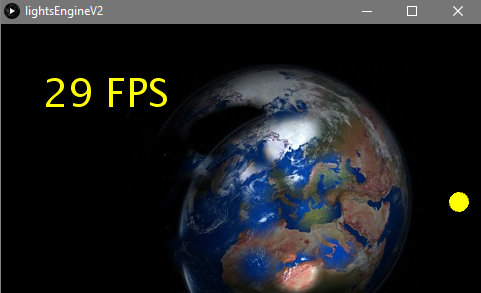#### Howdy, Stranger!

We are about to switch to a new forum software. Until then we have removed the registration on this forum.

# How to move with object...

edited October 2017

I want to move image with lights ... but lights doesnt moving ...Here is code:

``````PImage light, screen, bg, lightImage, mask;
int up, down, right, left;
float x, y, speed = 1;
color randomColor;
float camX=0, camY=0;
float  Ox1, Ox2, Oy1, Oy2;
int globalMX, globalMY;
void setup() {
size(480, 270, P3D);
frameRate(60);
background(0);
bg.resize(width, height);
light = bg.copy();
randomColor = color(random(256), random(256), random(256));
bullets = new ArrayList<Bullet>();
x = width/2;
y = height/2;
}
void draw() {
background(0);
x += (right - left) * speed;
y += (down - up) * speed;
Ox1=camX-width+x;
Ox2=camX+x;
Oy1=camY-y;
Oy2=camY+height-y;
ortho(Ox1, Ox2, Oy1, Oy2);
println(x + "  " + y);
imageMode(CORNER);
image(bg, 0, 0);
pushMatrix();
translate(x,y);
screen = get(0, 0, width, height);
popMatrix();
fill(0, 125);
noStroke();
rectMode(CENTER);
rect(x, y, width, height);
image(screen, 0,0);
fill(255, 255, 0);
textAlign(LEFT, TOP);
textSize(39);
text(int(frameRate) + " FPS", 5, 5);
ellipse(globalMX,globalMY,20,20);
//popMatrix();
}
pg.beginDraw();
pg.background(0);
for (int i = bullets.size()-1; i >= 0; i--) {
Bullet bullet = bullets.get(i);
drawLight(bullet.x, bullet.y, bullet.r);
}
pg.endDraw();
}
void drawLight(int xL, int yL, int rL) {
// pushMatrix();
// translate(x,y);
pg.imageMode(CENTER);
pg.image(lightImage, xL,yL, rL, rL);
//popMatrix();
}

void mousePressed() {
}
ArrayList<Bullet> bullets;
class Bullet {
int x, y, r;
public Bullet(int x_, int y_, int r_) {
x = x_;
y = y_;
r = r_;
}
}
void keyPressed() {
if (key == 'a' || key == 'A') {
left = 1;
}
if (key == 'd' || key == 'D') {
right = 1;
}
if (key == 'w' || key == 'W') {
up = 1;
}
if (key == 's' || key == 'S') {
down = 1;
}
}
void keyReleased() {
if (key == 'a' || key == 'A') {
left = 0;
}
if (key == 'd' || key == 'D') {
right = 0;
}
if (key == 'w' || key=='W') {
up = 0;
}
if (key == 's' || key == 'S') {
down = 0;
}
}
void mouseMoved() {
globalMX=mouseX;
globalMY=mouseY;
}
``````
Tagged:

• I guess jeremydouglass is the expert here...

• No, I think I'm done. I have asked @GeorgeJava to write more than a few words. I have asked many times. I'm tired of asking.

Move image how? Move light how? Move when, move which way? ...nobody knows.

• It’s hilarious

• (i think it's mostly a language thing. how is your czech?)

• Honestly, it just seems lazy -- Google Translate has Czech -> English .